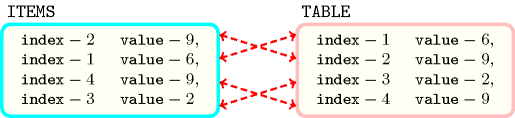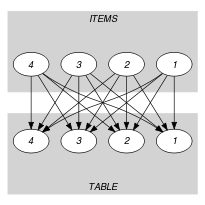## 5.147. elements_alldifferent

Origin
Constraint

$\mathrm{𝚎𝚕𝚎𝚖𝚎𝚗𝚝𝚜}_\mathrm{𝚊𝚕𝚕𝚍𝚒𝚏𝚏𝚎𝚛𝚎𝚗𝚝}\left(\mathrm{𝙸𝚃𝙴𝙼𝚂},\mathrm{𝚃𝙰𝙱𝙻𝙴}\right)$

Synonyms

$\mathrm{𝚎𝚕𝚎𝚖𝚎𝚗𝚝𝚜}_\mathrm{𝚊𝚕𝚕𝚍𝚒𝚏𝚏}$, $\mathrm{𝚎𝚕𝚎𝚖𝚎𝚗𝚝𝚜}_\mathrm{𝚊𝚕𝚕𝚍𝚒𝚜𝚝𝚒𝚗𝚌𝚝}$.

Arguments
 $\mathrm{𝙸𝚃𝙴𝙼𝚂}$ $\mathrm{𝚌𝚘𝚕𝚕𝚎𝚌𝚝𝚒𝚘𝚗}\left(\mathrm{𝚒𝚗𝚍𝚎𝚡}-\mathrm{𝚍𝚟𝚊𝚛},\mathrm{𝚟𝚊𝚕𝚞𝚎}-\mathrm{𝚍𝚟𝚊𝚛}\right)$ $\mathrm{𝚃𝙰𝙱𝙻𝙴}$ $\mathrm{𝚌𝚘𝚕𝚕𝚎𝚌𝚝𝚒𝚘𝚗}\left(\mathrm{𝚒𝚗𝚍𝚎𝚡}-\mathrm{𝚒𝚗𝚝},\mathrm{𝚟𝚊𝚕𝚞𝚎}-\mathrm{𝚍𝚟𝚊𝚛}\right)$
Restrictions
 $\mathrm{𝚛𝚎𝚚𝚞𝚒𝚛𝚎𝚍}$$\left(\mathrm{𝙸𝚃𝙴𝙼𝚂},\left[\mathrm{𝚒𝚗𝚍𝚎𝚡},\mathrm{𝚟𝚊𝚕𝚞𝚎}\right]\right)$ $\mathrm{𝙸𝚃𝙴𝙼𝚂}.\mathrm{𝚒𝚗𝚍𝚎𝚡}\ge 1$ $\mathrm{𝙸𝚃𝙴𝙼𝚂}.\mathrm{𝚒𝚗𝚍𝚎𝚡}\le |\mathrm{𝚃𝙰𝙱𝙻𝙴}|$ $|\mathrm{𝙸𝚃𝙴𝙼𝚂}|=|\mathrm{𝚃𝙰𝙱𝙻𝙴}|$ $\mathrm{𝚛𝚎𝚚𝚞𝚒𝚛𝚎𝚍}$$\left(\mathrm{𝚃𝙰𝙱𝙻𝙴},\left[\mathrm{𝚒𝚗𝚍𝚎𝚡},\mathrm{𝚟𝚊𝚕𝚞𝚎}\right]\right)$ $\mathrm{𝚃𝙰𝙱𝙻𝙴}.\mathrm{𝚒𝚗𝚍𝚎𝚡}\ge 1$ $\mathrm{𝚃𝙰𝙱𝙻𝙴}.\mathrm{𝚒𝚗𝚍𝚎𝚡}\le |\mathrm{𝚃𝙰𝙱𝙻𝙴}|$ $\mathrm{𝚍𝚒𝚜𝚝𝚒𝚗𝚌𝚝}$$\left(\mathrm{𝚃𝙰𝙱𝙻𝙴},\mathrm{𝚒𝚗𝚍𝚎𝚡}\right)$
Purpose

All the items of the $\mathrm{𝙸𝚃𝙴𝙼𝚂}$ collection should be equal to one of the entries of the table $\mathrm{𝚃𝙰𝙱𝙻𝙴}$ and all the variables $\mathrm{𝙸𝚃𝙴𝙼𝚂}.\mathrm{𝚒𝚗𝚍𝚎𝚡}$ should take distinct values.

Example
$\left(\begin{array}{c}〈\begin{array}{cc}\mathrm{𝚒𝚗𝚍𝚎𝚡}-2\hfill & \mathrm{𝚟𝚊𝚕𝚞𝚎}-9,\hfill \\ \mathrm{𝚒𝚗𝚍𝚎𝚡}-1\hfill & \mathrm{𝚟𝚊𝚕𝚞𝚎}-6,\hfill \\ \mathrm{𝚒𝚗𝚍𝚎𝚡}-4\hfill & \mathrm{𝚟𝚊𝚕𝚞𝚎}-9,\hfill \\ \mathrm{𝚒𝚗𝚍𝚎𝚡}-3\hfill & \mathrm{𝚟𝚊𝚕𝚞𝚎}-2\hfill \end{array}〉,\hfill \\ 〈\begin{array}{cc}\mathrm{𝚒𝚗𝚍𝚎𝚡}-1\hfill & \mathrm{𝚟𝚊𝚕𝚞𝚎}-6,\hfill \\ \mathrm{𝚒𝚗𝚍𝚎𝚡}-2\hfill & \mathrm{𝚟𝚊𝚕𝚞𝚎}-9,\hfill \\ \mathrm{𝚒𝚗𝚍𝚎𝚡}-3\hfill & \mathrm{𝚟𝚊𝚕𝚞𝚎}-2,\hfill \\ \mathrm{𝚒𝚗𝚍𝚎𝚡}-4\hfill & \mathrm{𝚟𝚊𝚕𝚞𝚎}-9\hfill \end{array}〉\hfill \end{array}\right)$

The $\mathrm{𝚎𝚕𝚎𝚖𝚎𝚗𝚝𝚜}_\mathrm{𝚊𝚕𝚕𝚍𝚒𝚏𝚏𝚎𝚛𝚎𝚗𝚝}$ constraint holds since, as depicted by Figure 5.147.1, there is a one to one correspondence between the items of the $\mathrm{𝙸𝚃𝙴𝙼𝚂}$ collection and the items of the $\mathrm{𝚃𝙰𝙱𝙻𝙴}$ collection.

##### Figure 5.147.1. Illustration of the one to one correspondence between the items of $\mathrm{𝙸𝚃𝙴𝙼𝚂}$ and the items of $\mathrm{𝚃𝙰𝙱𝙻𝙴}$Typical
 $|\mathrm{𝙸𝚃𝙴𝙼𝚂}|>1$ $\mathrm{𝚛𝚊𝚗𝚐𝚎}$$\left(\mathrm{𝙸𝚃𝙴𝙼𝚂}.\mathrm{𝚟𝚊𝚕𝚞𝚎}\right)>1$ $|\mathrm{𝚃𝙰𝙱𝙻𝙴}|>1$ $\mathrm{𝚛𝚊𝚗𝚐𝚎}$$\left(\mathrm{𝚃𝙰𝙱𝙻𝙴}.\mathrm{𝚟𝚊𝚕𝚞𝚎}\right)>1$
Symmetries
• Arguments are permutable w.r.t. permutation $\left(\mathrm{𝙸𝚃𝙴𝙼𝚂},\mathrm{𝚃𝙰𝙱𝙻𝙴}\right)$.

• Items of $\mathrm{𝙸𝚃𝙴𝙼𝚂}$ are permutable.

• Items of $\mathrm{𝚃𝙰𝙱𝙻𝙴}$ are permutable.

• All occurrences of two distinct values in $\mathrm{𝙸𝚃𝙴𝙼𝚂}.\mathrm{𝚟𝚊𝚕𝚞𝚎}$ or $\mathrm{𝚃𝙰𝙱𝙻𝙴}.\mathrm{𝚟𝚊𝚕𝚞𝚎}$ can be swapped; all occurrences of a value in $\mathrm{𝙸𝚃𝙴𝙼𝚂}.\mathrm{𝚟𝚊𝚕𝚞𝚎}$ or $\mathrm{𝚃𝙰𝙱𝙻𝙴}.\mathrm{𝚟𝚊𝚕𝚞𝚎}$ can be renamed to any unused value.

Arg. properties

Functional dependency: $\mathrm{𝙸𝚃𝙴𝙼𝚂}.\mathrm{𝚟𝚊𝚕𝚞𝚎}$ determined by $\mathrm{𝙸𝚃𝙴𝙼𝚂}.\mathrm{𝚒𝚗𝚍𝚎𝚡}$ and $\mathrm{𝚃𝙰𝙱𝙻𝙴}$.

Usage

Used for replacing by a single $\mathrm{𝚎𝚕𝚎𝚖𝚎𝚗𝚝𝚜}_\mathrm{𝚊𝚕𝚕𝚍𝚒𝚏𝚏𝚎𝚛𝚎𝚗𝚝}$ constraint an $\mathrm{𝚊𝚕𝚕𝚍𝚒𝚏𝚏𝚎𝚛𝚎𝚗𝚝}$ and a set of $\mathrm{𝚎𝚕𝚎𝚖𝚎𝚗𝚝}$ constraints having the following structure:

For instance, the constraint given in the Example slot is equivalent to the conjunction of the following set of constraints:

$\mathrm{𝚊𝚕𝚕𝚍𝚒𝚏𝚏𝚎𝚛𝚎𝚗𝚝}\left(〈\mathrm{𝚟𝚊𝚛}-2,\mathrm{𝚟𝚊𝚛}-1,\mathrm{𝚟𝚊𝚛}-4,\mathrm{𝚟𝚊𝚛}-3〉\right)$
$\mathrm{𝚎𝚕𝚎𝚖𝚎𝚗𝚝}\left(\begin{array}{c}〈\begin{array}{cc}\mathrm{𝚒𝚗𝚍𝚎𝚡}-2\hfill & \mathrm{𝚟𝚊𝚕𝚞𝚎}-9\hfill \end{array}〉,\hfill \\ 〈\begin{array}{cc}\mathrm{𝚒𝚗𝚍𝚎𝚡}-1\hfill & \mathrm{𝚟𝚊𝚕𝚞𝚎}-6,\hfill \\ \mathrm{𝚒𝚗𝚍𝚎𝚡}-2\hfill & \mathrm{𝚟𝚊𝚕𝚞𝚎}-9,\hfill \\ \mathrm{𝚒𝚗𝚍𝚎𝚡}-3\hfill & \mathrm{𝚟𝚊𝚕𝚞𝚎}-2,\hfill \\ \mathrm{𝚒𝚗𝚍𝚎𝚡}-4\hfill & \mathrm{𝚟𝚊𝚕𝚞𝚎}-9\hfill \end{array}〉\hfill \end{array}\right)$
$\mathrm{𝚎𝚕𝚎𝚖𝚎𝚗𝚝}\left(\begin{array}{c}〈\begin{array}{cc}\mathrm{𝚒𝚗𝚍𝚎𝚡}-1\hfill & \mathrm{𝚟𝚊𝚕𝚞𝚎}-6\hfill \end{array}〉,\hfill \\ 〈\begin{array}{cc}\mathrm{𝚒𝚗𝚍𝚎𝚡}-1\hfill & \mathrm{𝚟𝚊𝚕𝚞𝚎}-6,\hfill \\ \mathrm{𝚒𝚗𝚍𝚎𝚡}-2\hfill & \mathrm{𝚟𝚊𝚕𝚞𝚎}-9,\hfill \\ \mathrm{𝚒𝚗𝚍𝚎𝚡}-3\hfill & \mathrm{𝚟𝚊𝚕𝚞𝚎}-2,\hfill \\ \mathrm{𝚒𝚗𝚍𝚎𝚡}-4\hfill & \mathrm{𝚟𝚊𝚕𝚞𝚎}-9\hfill \end{array}〉\hfill \end{array}\right)$
$\mathrm{𝚎𝚕𝚎𝚖𝚎𝚗𝚝}\left(\begin{array}{c}〈\begin{array}{cc}\mathrm{𝚒𝚗𝚍𝚎𝚡}-3\hfill & \mathrm{𝚟𝚊𝚕𝚞𝚎}-2\hfill \end{array}〉,\hfill \\ 〈\begin{array}{cc}\mathrm{𝚒𝚗𝚍𝚎𝚡}-1\hfill & \mathrm{𝚟𝚊𝚕𝚞𝚎}-6,\hfill \\ \mathrm{𝚒𝚗𝚍𝚎𝚡}-2\hfill & \mathrm{𝚟𝚊𝚕𝚞𝚎}-9,\hfill \\ \mathrm{𝚒𝚗𝚍𝚎𝚡}-3\hfill & \mathrm{𝚟𝚊𝚕𝚞𝚎}-2,\hfill \\ \mathrm{𝚒𝚗𝚍𝚎𝚡}-4\hfill & \mathrm{𝚟𝚊𝚕𝚞𝚎}-9\hfill \end{array}〉\hfill \end{array}\right)$
$\mathrm{𝚎𝚕𝚎𝚖𝚎𝚗𝚝}\left(\begin{array}{c}〈\begin{array}{cc}\mathrm{𝚒𝚗𝚍𝚎𝚡}-4\hfill & \mathrm{𝚟𝚊𝚕𝚞𝚎}-9\hfill \end{array}〉,\hfill \\ 〈\begin{array}{cc}\mathrm{𝚒𝚗𝚍𝚎𝚡}-1\hfill & \mathrm{𝚟𝚊𝚕𝚞𝚎}-6,\hfill \\ \mathrm{𝚒𝚗𝚍𝚎𝚡}-2\hfill & \mathrm{𝚟𝚊𝚕𝚞𝚎}-9,\hfill \\ \mathrm{𝚒𝚗𝚍𝚎𝚡}-3\hfill & \mathrm{𝚟𝚊𝚕𝚞𝚎}-2,\hfill \\ \mathrm{𝚒𝚗𝚍𝚎𝚡}-4\hfill & \mathrm{𝚟𝚊𝚕𝚞𝚎}-9\hfill \end{array}〉\hfill \end{array}\right)$

As a practical example of utilisation of the $\mathrm{𝚎𝚕𝚎𝚖𝚎𝚗𝚝𝚜}_\mathrm{𝚊𝚕𝚕𝚍𝚒𝚏𝚏𝚎𝚛𝚎𝚗𝚝}$ constraint we show how to model the link between a permutation consisting of a single cycle and its expanded form. For instance, to the permutation $3,6,5,2,4,1$ corresponds the sequence $354261$. Let us note ${S}_{1},{S}_{2},{S}_{3},{S}_{4},{S}_{5},{S}_{6}$ the permutation and ${V}_{1}{V}_{2}{V}_{3}{V}_{4}{V}_{5}{V}_{6}$ its expanded form (see Figure 5.147.2).

The constraint:

$\mathrm{𝚎𝚕𝚎𝚖𝚎𝚗𝚝𝚜}_\mathrm{𝚊𝚕𝚕𝚍𝚒𝚏𝚏𝚎𝚛𝚎𝚗𝚝}\left(\begin{array}{c}〈\begin{array}{cc}\mathrm{𝚒𝚗𝚍𝚎𝚡}-{V}_{1}\hfill & \mathrm{𝚟𝚊𝚕𝚞𝚎}-{V}_{2},\hfill \\ \mathrm{𝚒𝚗𝚍𝚎𝚡}-{V}_{2}\hfill & \mathrm{𝚟𝚊𝚕𝚞𝚎}-{V}_{3},\hfill \\ \mathrm{𝚒𝚗𝚍𝚎𝚡}-{V}_{3}\hfill & \mathrm{𝚟𝚊𝚕𝚞𝚎}-{V}_{4},\hfill \\ \mathrm{𝚒𝚗𝚍𝚎𝚡}-{V}_{4}\hfill & \mathrm{𝚟𝚊𝚕𝚞𝚎}-{V}_{5},\hfill \\ \mathrm{𝚒𝚗𝚍𝚎𝚡}-{V}_{5}\hfill & \mathrm{𝚟𝚊𝚕𝚞𝚎}-{V}_{6},\hfill \\ \mathrm{𝚒𝚗𝚍𝚎𝚡}-{V}_{6}\hfill & \mathrm{𝚟𝚊𝚕𝚞𝚎}-{V}_{1}\hfill \end{array}〉,\hfill \\ 〈\begin{array}{cc}\mathrm{𝚒𝚗𝚍𝚎𝚡}-1\hfill & \mathrm{𝚟𝚊𝚕𝚞𝚎}-{S}_{1},\hfill \\ \mathrm{𝚒𝚗𝚍𝚎𝚡}-2\hfill & \mathrm{𝚟𝚊𝚕𝚞𝚎}-{S}_{2},\hfill \\ \mathrm{𝚒𝚗𝚍𝚎𝚡}-3\hfill & \mathrm{𝚟𝚊𝚕𝚞𝚎}-{S}_{3},\hfill \\ \mathrm{𝚒𝚗𝚍𝚎𝚡}-4\hfill & \mathrm{𝚟𝚊𝚕𝚞𝚎}-{S}_{4},\hfill \\ \mathrm{𝚒𝚗𝚍𝚎𝚡}-5\hfill & \mathrm{𝚟𝚊𝚕𝚞𝚎}-{S}_{5},\hfill \\ \mathrm{𝚒𝚗𝚍𝚎𝚡}-6\hfill & \mathrm{𝚟𝚊𝚕𝚞𝚎}-{S}_{6}\hfill \end{array}〉\hfill \end{array}\right)$

models the fact that ${S}_{1},{S}_{2},{S}_{3},{S}_{4},{S}_{5},{S}_{6}$ corresponds to a permutation with a single cycle. It also expresses the link between the variables ${S}_{1},{S}_{2},{S}_{3},{S}_{4},{S}_{5},{S}_{6}$ and ${V}_{1},{V}_{2},{V}_{3},{V}_{4},{V}_{5},{V}_{6}$.

##### Figure 5.147.2. Two representations of a permutation containing a single cycleReformulation

The $\mathrm{𝚎𝚕𝚎𝚖𝚎𝚗𝚝𝚜}_\mathrm{𝚊𝚕𝚕𝚍𝚒𝚏𝚏𝚎𝚛𝚎𝚗𝚝}$$\left(〈\mathrm{𝚒𝚗𝚍𝚎𝚡}-{I}_{1}\mathrm{𝚟𝚊𝚕𝚞𝚎}-{V}_{1},\mathrm{𝚒𝚗𝚍𝚎𝚡}-{I}_{2}\mathrm{𝚟𝚊𝚕𝚞𝚎}-{V}_{2},\cdots ,\mathrm{𝚒𝚗𝚍𝚎𝚡}-{I}_{|\mathrm{𝙸𝚃𝙴𝙼𝚂}|}\mathrm{𝚟𝚊𝚕𝚞𝚎}-{V}_{|\mathrm{𝙸𝚃𝙴𝙼𝚂}|}〉,\mathrm{𝚃𝙰𝙱𝙻𝙴}\right)$ constraint can be expressed in term of a conjunction of $|\mathrm{𝙸𝚃𝙴𝙼𝚂}|$ $\mathrm{𝚎𝚕𝚎𝚖}$ constraints and of one $\mathrm{𝚊𝚕𝚕𝚍𝚒𝚏𝚏𝚎𝚛𝚎𝚗𝚝}$ constraint of the form:

$\mathrm{𝚎𝚕𝚎𝚖}$$\left(〈\mathrm{𝚒𝚗𝚍𝚎𝚡}-{I}_{1}\mathrm{𝚟𝚊𝚕𝚞𝚎}-{V}_{1}〉,\mathrm{𝚃𝙰𝙱𝙻𝙴}\right)$,

$\mathrm{𝚎𝚕𝚎𝚖}$$\left(〈\mathrm{𝚒𝚗𝚍𝚎𝚡}-{I}_{2}\mathrm{𝚟𝚊𝚕𝚞𝚎}-{V}_{2}〉,\mathrm{𝚃𝙰𝙱𝙻𝙴}\right)$,

$\cdots$

$\mathrm{𝚎𝚕𝚎𝚖}$$\left(〈\mathrm{𝚒𝚗𝚍𝚎𝚡}-{I}_{|\mathrm{𝙸𝚃𝙴𝙼𝚂}|}\mathrm{𝚟𝚊𝚕𝚞𝚎}-{V}_{|\mathrm{𝙸𝚃𝙴𝙼𝚂}|}〉,\mathrm{𝚃𝙰𝙱𝙻𝙴}\right)$,

$\mathrm{𝚊𝚕𝚕𝚍𝚒𝚏𝚏𝚎𝚛𝚎𝚗𝚝}$$\left(〈{I}_{1},{I}_{2},\cdots ,{I}_{|\mathrm{𝙸𝚃𝙴𝙼𝚂}|}〉\right)$.

Keywords
Cond. implications

$\mathrm{𝚎𝚕𝚎𝚖𝚎𝚗𝚝𝚜}_\mathrm{𝚊𝚕𝚕𝚍𝚒𝚏𝚏𝚎𝚛𝚎𝚗𝚝}\left(\mathrm{𝙸𝚃𝙴𝙼𝚂},\mathrm{𝚃𝙰𝙱𝙻𝙴}\right)$

with  $\mathrm{𝚃𝙰𝙱𝙻𝙴}.\mathrm{𝚟𝚊𝚕𝚞𝚎}\ge 0$

implies $\mathrm{𝚋𝚒𝚗}_\mathrm{𝚙𝚊𝚌𝚔𝚒𝚗𝚐}_\mathrm{𝚌𝚊𝚙𝚊}$$\left(\mathrm{𝚃𝙰𝙱𝙻𝙴},\mathrm{𝙸𝚃𝙴𝙼𝚂}\right)$.

Arc input(s)

$\mathrm{𝙸𝚃𝙴𝙼𝚂}$ $\mathrm{𝚃𝙰𝙱𝙻𝙴}$

Arc generator
$\mathrm{𝑃𝑅𝑂𝐷𝑈𝐶𝑇}$$↦\mathrm{𝚌𝚘𝚕𝚕𝚎𝚌𝚝𝚒𝚘𝚗}\left(\mathrm{𝚒𝚝𝚎𝚖𝚜},\mathrm{𝚝𝚊𝚋𝚕𝚎}\right)$

Arc arity
Arc constraint(s)
 $•\mathrm{𝚒𝚝𝚎𝚖𝚜}.\mathrm{𝚒𝚗𝚍𝚎𝚡}=\mathrm{𝚝𝚊𝚋𝚕𝚎}.\mathrm{𝚒𝚗𝚍𝚎𝚡}$ $•\mathrm{𝚒𝚝𝚎𝚖𝚜}.\mathrm{𝚟𝚊𝚕𝚞𝚎}=\mathrm{𝚝𝚊𝚋𝚕𝚎}.\mathrm{𝚟𝚊𝚕𝚞𝚎}$
Graph property(ies)
$\mathrm{𝐍𝐕𝐄𝐑𝐓𝐄𝐗}$$=|\mathrm{𝙸𝚃𝙴𝙼𝚂}|+|\mathrm{𝚃𝙰𝙱𝙻𝙴}|$

Graph model

The fact that all variables $\mathrm{𝙸𝚃𝙴𝙼𝚂}.\mathrm{𝚒𝚗𝚍𝚎𝚡}$ are pairwise different is derived from the conjunctions of the following facts:

• From the graph property $\mathrm{𝐍𝐕𝐄𝐑𝐓𝐄𝐗}=|\mathrm{𝙸𝚃𝙴𝙼𝚂}|+|\mathrm{𝚃𝙰𝙱𝙻𝙴}|$ it follows that all vertices of the initial graph belong also to the final graph,

• A vertex $v$ belongs to the final graph if there is at least one constraint involving $v$ that holds,

• From the first condition $\mathrm{𝚒𝚝𝚎𝚖𝚜}.\mathrm{𝚒𝚗𝚍𝚎𝚡}=\mathrm{𝚝𝚊𝚋𝚕𝚎}.\mathrm{𝚒𝚗𝚍𝚎𝚡}$ of the arc constraint, and from the restriction $\mathrm{𝚍𝚒𝚜𝚝𝚒𝚗𝚌𝚝}\left(\mathrm{𝚃𝙰𝙱𝙻𝙴}.\mathrm{𝚒𝚗𝚍𝚎𝚡}\right)$ it follows: for all vertices $v$ generated from the collection $\mathrm{𝙸𝚃𝙴𝙼𝚂}$ at most one constraint involving $v$ holds.

Parts (A) and (B) of Figure 5.147.3 respectively show the initial and final graph associated with the Example slot. Since we use the $\mathrm{𝐍𝐕𝐄𝐑𝐓𝐄𝐗}$ graph property, the vertices of the final graph are stressed in bold.

##### Figure 5.147.3. Initial and final graph of the $\mathrm{𝚎𝚕𝚎𝚖𝚎𝚗𝚝𝚜}_\mathrm{𝚊𝚕𝚕𝚍𝚒𝚏𝚏𝚎𝚛𝚎𝚗𝚝}$ constraint(a) (b)
Signature

Since the final graph cannot have more than $|\mathrm{𝙸𝚃𝙴𝙼𝚂}|+|\mathrm{𝚃𝙰𝙱𝙻𝙴}|$ vertices one can simplify $\underline{\overline{\mathrm{𝐍𝐕𝐄𝐑𝐓𝐄𝐗}}}$ to $\overline{\mathrm{𝐍𝐕𝐄𝐑𝐓𝐄𝐗}}$.